Скачать презентацию Free-Standing Mathematics Activity Maximising and minimising Nuffield

6a9febd53deb5d2341612768bf781d2f.ppt

• Количество слайдов: 18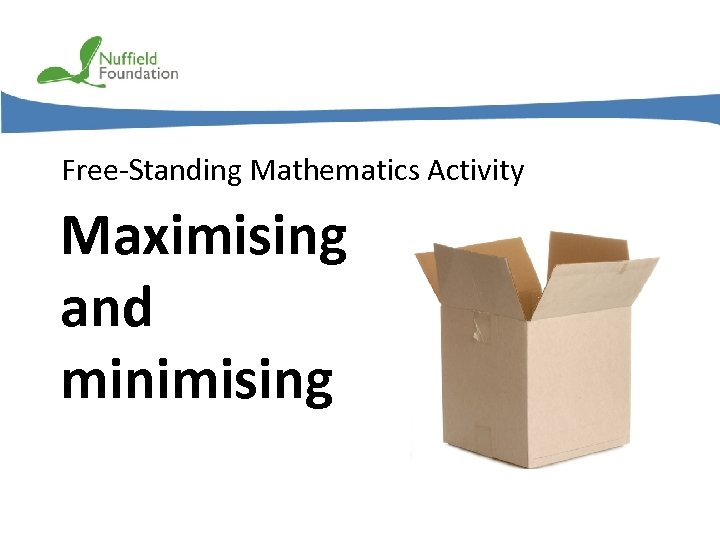Free-Standing Mathematics Activity Maximising and minimising © Nuffield Foundation 2011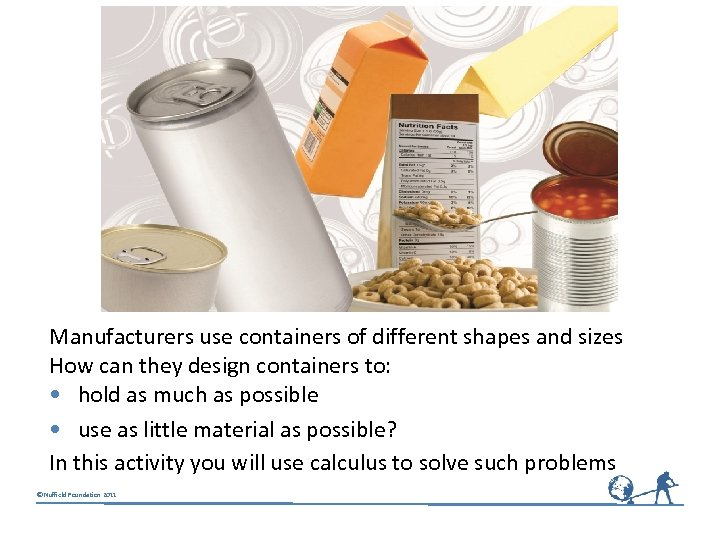Manufacturers use containers of different shapes and sizes How can they design containers to: • hold as much as possible • use as little material as possible? In this activity you will use calculus to solve such problems © Nuffield Foundation 2011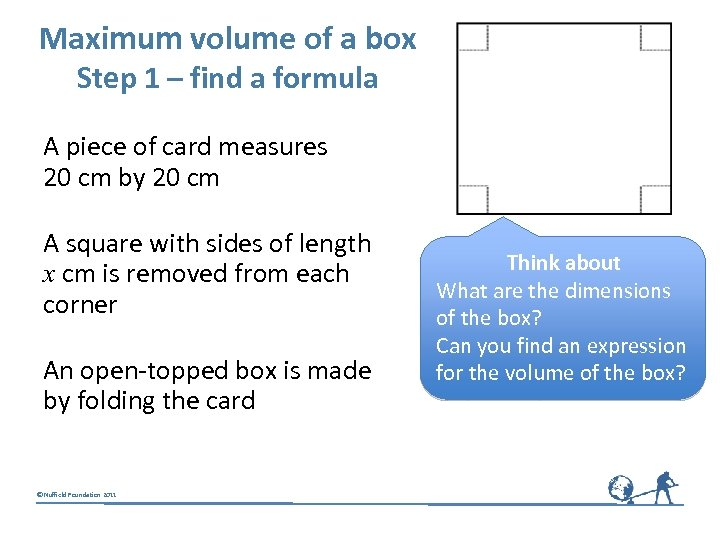Maximum volume of a box Step 1 – find a formula A piece of card measures 20 cm by 20 cm A square with sides of length x cm is removed from each corner An open-topped box is made by folding the card © Nuffield Foundation 2011 Think about What are the dimensions of the box? Can you find an expression for the volume of the box?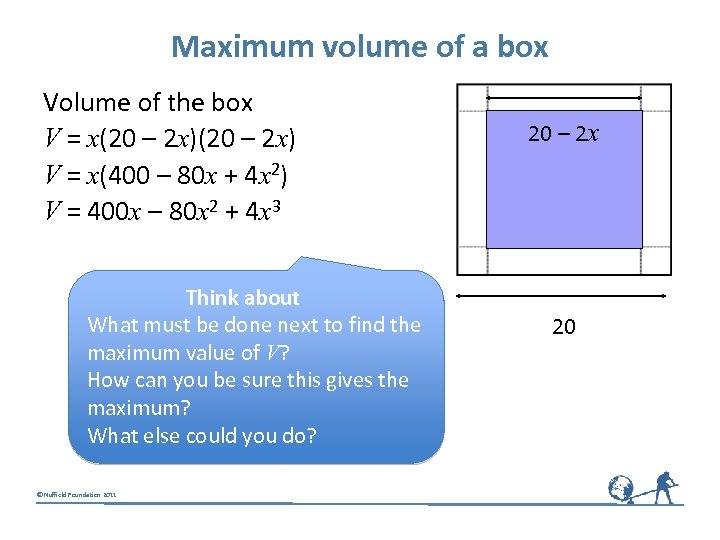Maximum volume of a box Volume of the box V = x(20 – 2 x) V = x(400 – 80 x + 4 x 2) V = 400 x – 80 x 2 + 4 x 3 Think about What must be done next to find the maximum value of V? How can you be sure this gives the maximum? What else could you do? © Nuffield Foundation 2011 20 – 2 x 20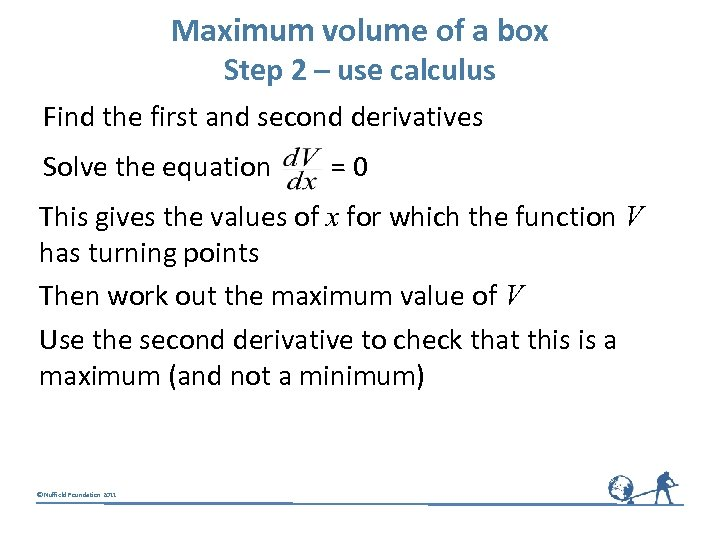Maximum volume of a box Step 2 – use calculus Find the first and second derivatives Solve the equation = 0 This gives the values of x for which the function V has turning points Then work out the maximum value of V Use the second derivative to check that this is a maximum (and not a minimum) © Nuffield Foundation 2011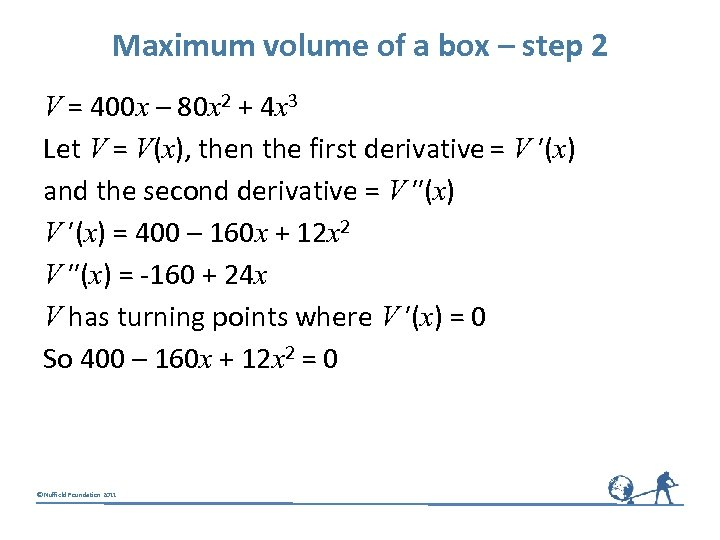Maximum volume of a box – step 2 V = 400 x – 80 x 2 + 4 x 3 Let V = V(x), then the first derivative = V ′(x) and the second derivative = V ″(x) V ′(x) = 400 – 160 x + 12 x 2 V ″(x) = -160 + 24 x V has turning points where V ′(x) = 0 So 400 – 160 x + 12 x 2 = 0 © Nuffield Foundation 2011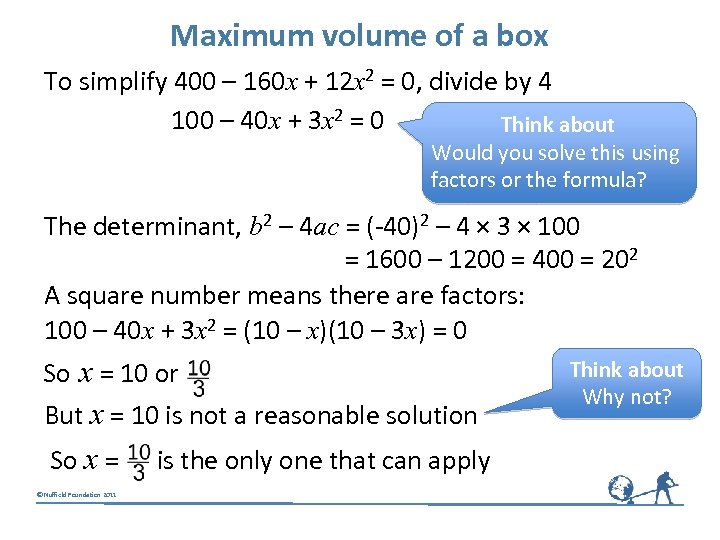Maximum volume of a box To simplify 400 – 160 x + 12 x 2 = 0, divide by 4 100 – 40 x + 3 x 2 = 0 Think about Would you solve this using factors or the formula? The determinant, b 2 – 4 ac = (-40)2 – 4 × 3 × 100 = 1600 – 1200 = 400 = 202 A square number means there are factors: 100 – 40 x + 3 x 2 = (10 – x)(10 – 3 x) = 0 So x = 10 or But x = 10 is not a reasonable solution So x = is the only one that can apply © Nuffield Foundation 2011 Think about Why not?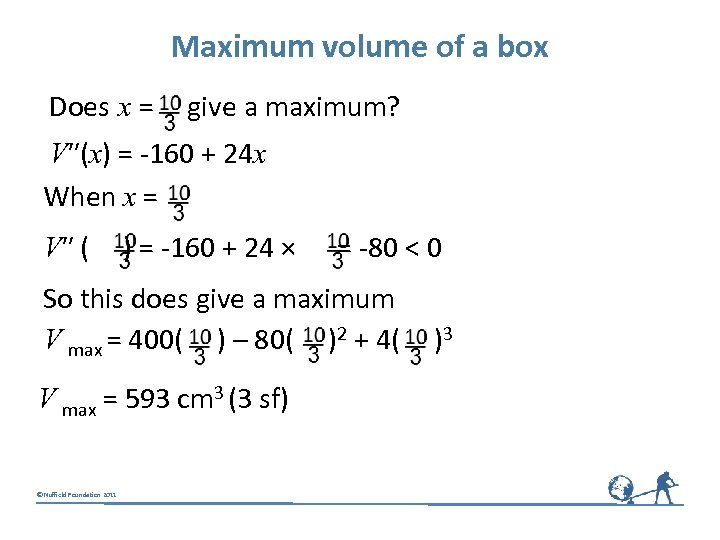Maximum volume of a box Does x = give a maximum? V″(x) = -160 + 24 x When x = V″ ( ) = -160 + 24 × = -80 < 0 So this does give a maximum V max = 400( ) – 80( )2 + 4( )3 V max = 593 cm 3 (3 sf) © Nuffield Foundation 2011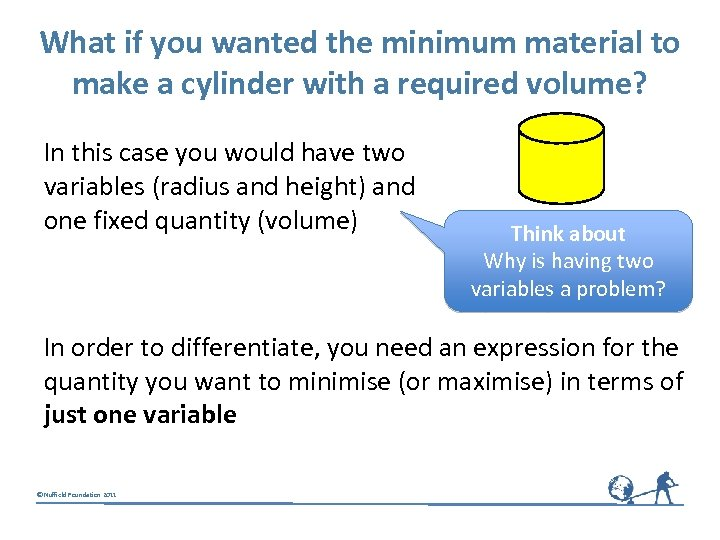What if you wanted the minimum material to make a cylinder with a required volume? In this case you would have two variables (radius and height) and one fixed quantity (volume) Think about Why is having two variables a problem? In order to differentiate, you need an expression for the quantity you want to minimise (or maximise) in terms of just one variable © Nuffield Foundation 2011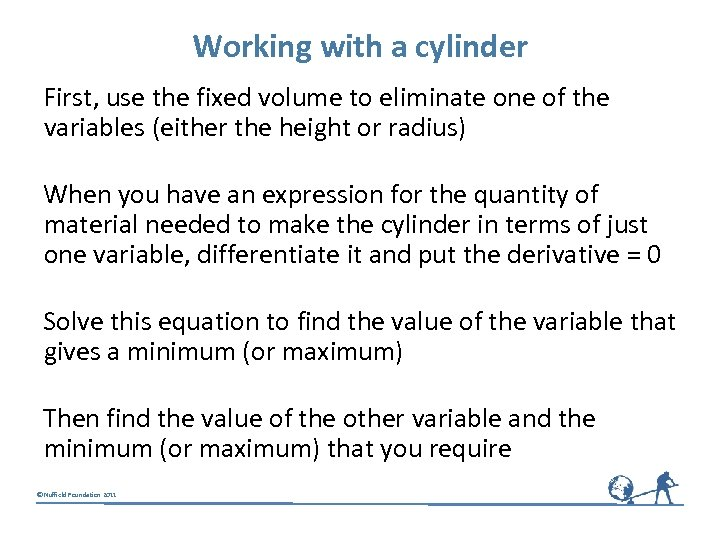Working with a cylinder First, use the fixed volume to eliminate one of the variables (either the height or radius) When you have an expression for the quantity of material needed to make the cylinder in terms of just one variable, differentiate it and put the derivative = 0 Solve this equation to find the value of the variable that gives a minimum (or maximum) Then find the value of the other variable and the minimum (or maximum) that you require © Nuffield Foundation 2011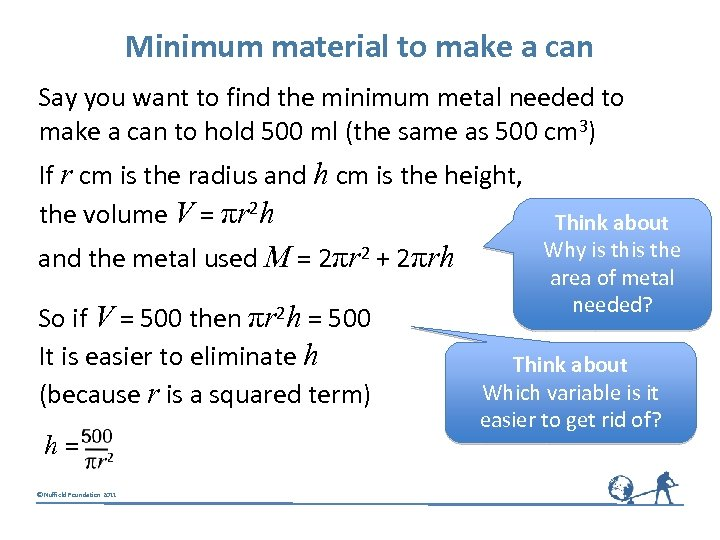Minimum material to make a can Say you want to find the minimum metal needed to make a can to hold 500 ml (the same as 500 cm 3) If r cm is the radius and h cm is the height, the volume V = πr 2 h and the metal used M = 2πr 2 + 2πrh So if V = 500 then πr 2 h = 500 It is easier to eliminate h (because r is a squared term) h = © Nuffield Foundation 2011 Think about Why is the area of metal needed? Think about Which variable is it easier to get rid of?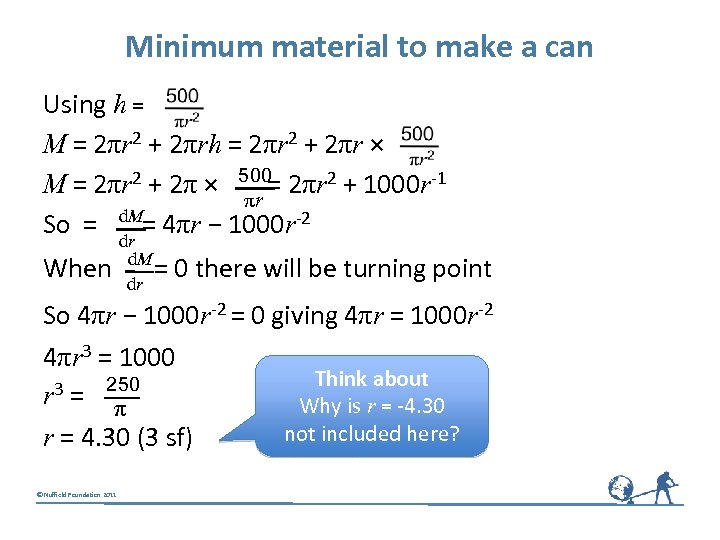Minimum material to make a can Using h = M = 2πr 2 + 2πrh = 2πr 2 + 2πr × 500 M = 2πr 2 + 2π × = 2πr 2 + 1000 r-1 πr d. M So = = 4πr − 1000 r-2 dr d. M When = 0 there will be turning point dr So 4πr − 1000 r-2 = 0 giving 4πr = 1000 r-2 4πr 3 = 1000 r 3 = 250 π r = 4. 30 (3 sf) © Nuffield Foundation 2011 Think about Why is r = -4. 30 not included here?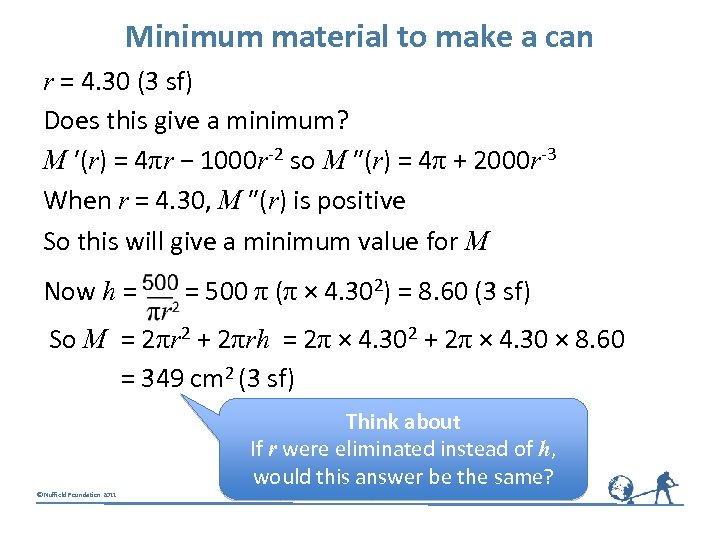Minimum material to make a can r = 4. 30 (3 sf) Does this give a minimum? M ′(r) = 4πr − 1000 r-2 so M ″(r) = 4π + 2000 r-3 When r = 4. 30, M ″(r) is positive So this will give a minimum value for M Now h = = 500 π (π × 4. 302) = 8. 60 (3 sf) So M = 2πr 2 + 2πrh = 2π × 4. 302 + 2π × 4. 30 × 8. 60 = 349 cm 2 (3 sf) Think about If r were eliminated instead of h, would this answer be the same? © Nuffield Foundation 2011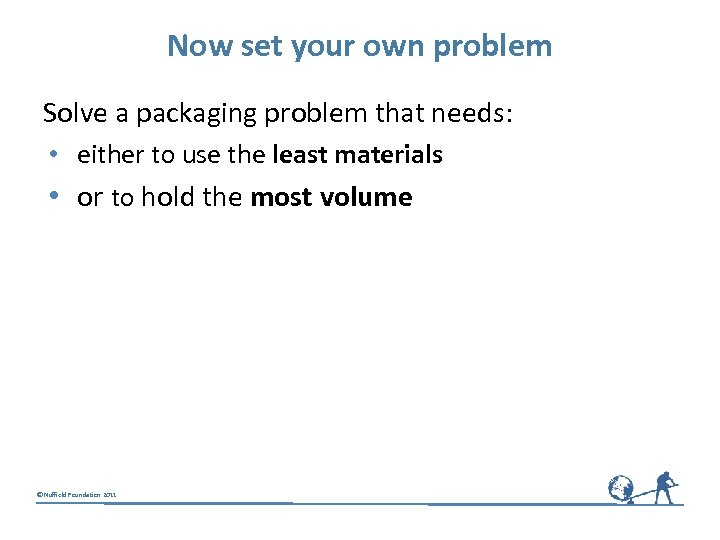Now set your own problem Solve a packaging problem that needs: • either to use the least materials • or to hold the most volume © Nuffield Foundation 2011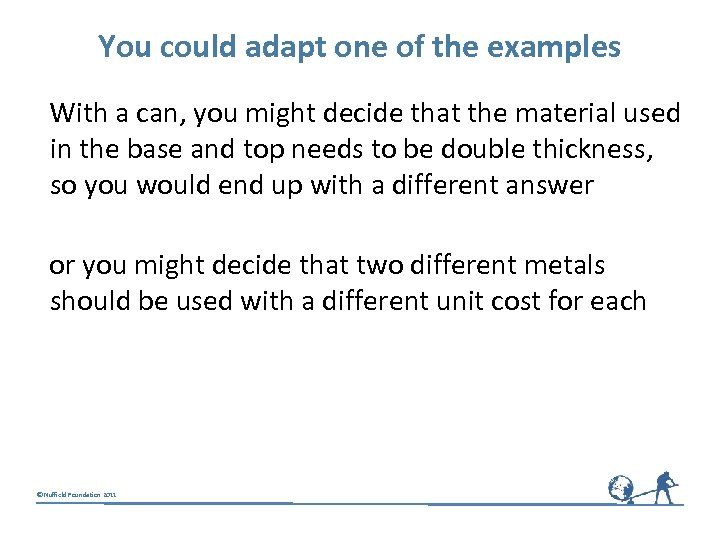You could adapt one of the examples With a can, you might decide that the material used in the base and top needs to be double thickness, so you would end up with a different answer or you might decide that two different metals should be used with a different unit cost for each © Nuffield Foundation 2011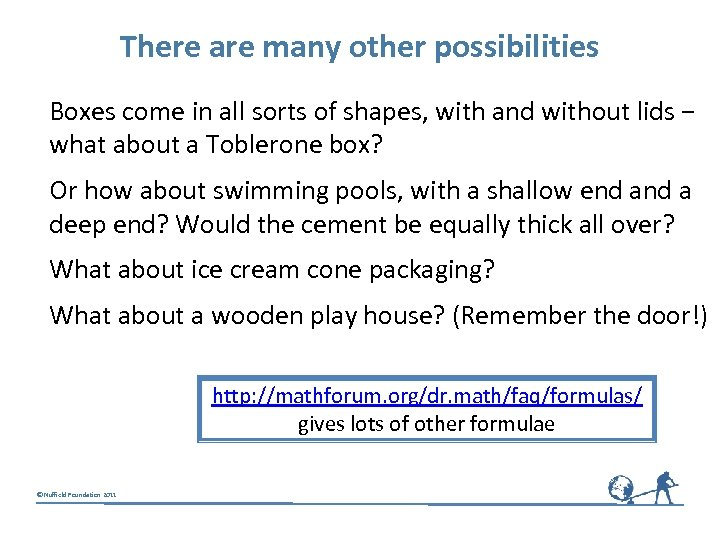There are many other possibilities Boxes come in all sorts of shapes, with and without lids − what about a Toblerone box? Or how about swimming pools, with a shallow end a deep end? Would the cement be equally thick all over? What about ice cream cone packaging? What about a wooden play house? (Remember the door!) http: //mathforum. org/dr. math/faq/formulas/ gives lots of other formulae © Nuffield Foundation 2011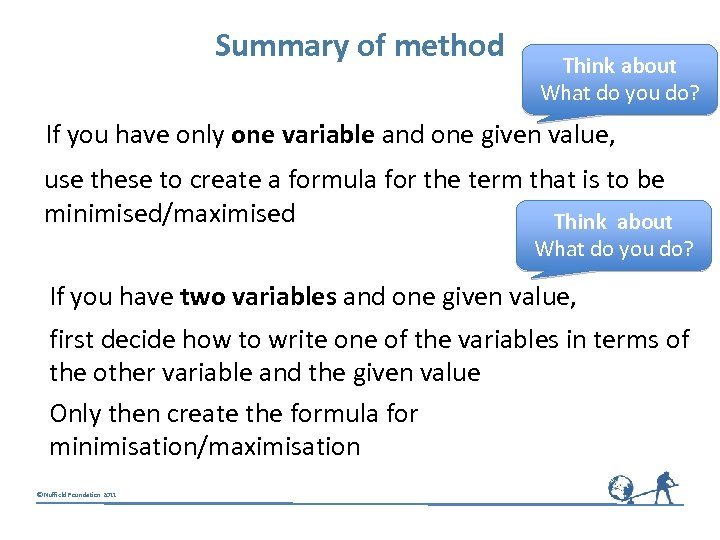Summary of method Think about What do you do? If you have only one variable and one given value, use these to create a formula for the term that is to be minimised/maximised Think about What do you do? If you have two variables and one given value, first decide how to write one of the variables in terms of the other variable and the given value Only then create the formula for minimisation/maximisation © Nuffield Foundation 2011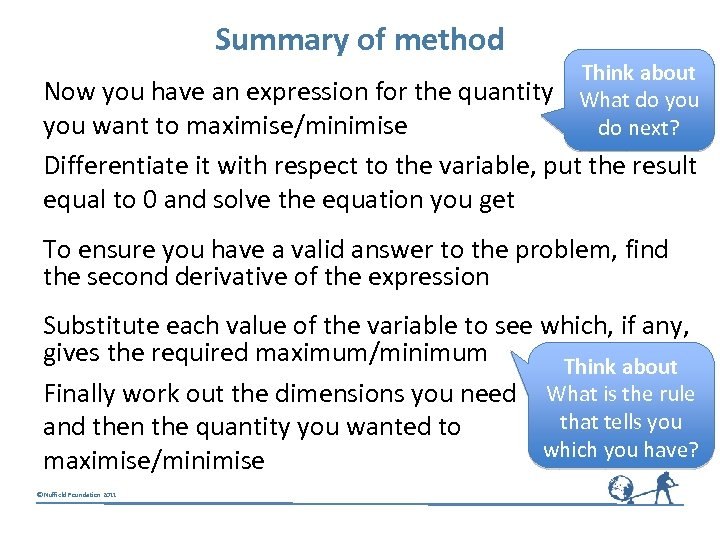Summary of method Think about What do you do next? Now you have an expression for the quantity you want to maximise/minimise Differentiate it with respect to the variable, put the result equal to 0 and solve the equation you get To ensure you have a valid answer to the problem, find the second derivative of the expression Substitute each value of the variable to see which, if any, gives the required maximum/minimum Think about Finally work out the dimensions you need What is the rule that tells you and then the quantity you wanted to which you have? maximise/minimise © Nuffield Foundation 2011